Chemistry

## Quiz 6 : Purification and Characterization of Triacylglycerols in Natural OilsLooking for Biochemistry Homework Help?# Quiz 6 : Purification and Characterization of Triacylglycerols in Natural Oils

In this problem, we are asked to calculate the half-life of an isotope from data - at t = 0, the activity is 300 disintegrations per minute. At t = 60 minutes, the activity is 200 disintegrations per minute. The half-life is the time required to lose one-half of the activity. By extension, in this problem it will be the time required to go from 300 disintegrations to 150 disintegrations per minute. We will calculate ? , the decay constant, and then use this to calculate our half-life, as follows: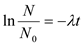Where: N number of disintegrations at t N 0 number of disintegrations at t=0 T the time And half-life is equal to: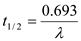Thus, our ? is calculated as follows: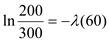? equals 0.00676. And our half-life equals: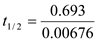Or 102.5 - our original problem had only one significant figure, though, so we would record our half-life as 100 minutes.

In this problem, we are asked to calculate the decay constant for phosphorus-32, 32 P. The decay constant, denoted ? , is a proportionality constant that is used to figure the speeds at which different atoms radioactively decay. The equation used to determine this requires knowledge of the half-life ( t 1/2 ) of the atom, and is as follows:The half-life of 32 P is 14.2 days, and therefore we would calculate ? as follows:Therefore, ? = 0.049 day -1.

In this problem, we are asked to explain how we can tell that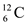is a stable isotope. Very simply, stable isotopes of atoms will have the same number of protons and neutrons; this atom of carbon-12 has six protons (as defined by its atomic number) and must also have six neutrons (to give it a mass number of 12). This rule breaks down for atoms of atomic number 84 and above.